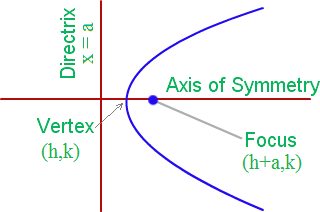# Parabola Formula

A set of points on a plain surface that forms a curve such that any point on the curve is at equidistant from the focus is a parabola. One of the properties of parabolas is that they are made of a material that reflects light that travels parallel to the axis of symmetry of a parabola and strikes its concave side which is reflected its focus.

The line that runs down the parabola’s centre and passes through the focus and vertex of the parabola and perpendicular to its directrix is the axis. It divides the graph into two equal parts.

Vertex is the coordinate from the parabola takes its sharpest turn. It is that point at which the parabola intersects the axis and cannot go any higher or lower in a coordinate plane.

The straight line that is used to generate a curve is directrix. This is the line from which the parabola curves away. The directrix is perpendicular to the axis of symmetry.The Parabola Formula for the equation of a parabola given in its standard form, y = ax2 + bx + c is given below:

$\large Verte\;of\;the\;Parabola=\frac{-b}{2a}, \frac{4ac-b^{2}}{4a}$

$\large Focus\;of\;the\;Parabola=\left(\frac{-b}{2a}, \frac{4ac-b^{2}+1}{4a}\right)$

$$\begin{array}{l} \large Directrix\ of\ Parabola\ y=\frac{4ac-b^{2}-1}{4a}\end{array}$$

### Solved Examples

Question: Find the vertex, focus and directrix of a parabola of equation y = 5x2 + 3x + 2.

Solution:

Given,
y = 5x2 + 3x + 2
Comparing the above equation with the general form of parabola equation y = ax2 + bx + c we get,
a = 5
b = 3
c = 2

Vertex of a parabola

= (

$$\begin{array}{l}\frac{-b}{2a}\end{array}$$
,
$$\begin{array}{l}\frac{4ac-b^{2}}{4a}\end{array}$$
)

= (

$$\begin{array}{l}\frac{-3}{2(5)}\end{array}$$
,
$$\begin{array}{l}\frac{4(5)(2)-3^{2}}{4(5)}\end{array}$$
)

= (

$$\begin{array}{l}\frac{-3}{10}\end{array}$$
,
$$\begin{array}{l}\frac{40-9}{20}\end{array}$$
)

= (

$$\begin{array}{l}\frac{-3}{10}\end{array}$$
,
$$\begin{array}{l}\frac{31}{20}\end{array}$$
)

Focus of a parabola

= (

$$\begin{array}{l}\frac{-b}{2a}\end{array}$$
,
$$\begin{array}{l}\frac{4ac-b^{2}+1}{4a}\end{array}$$
)

= (

$$\begin{array}{l}\frac{-3}{2(5)}\end{array}$$
,
$$\begin{array}{l}\frac{4(5)(2)-3^{2}+1}{4(5)}\end{array}$$
)

= (

$$\begin{array}{l}\frac{-3}{10}\end{array}$$
,
$$\begin{array}{l}\frac{40-9+1}{20}\end{array}$$
)

= (

$$\begin{array}{l}\frac{-3}{10}\end{array}$$
,
$$\begin{array}{l}\frac{32}{20}\end{array}$$
)

= (

$$\begin{array}{l}\frac{-3}{10}\end{array}$$
,
$$\begin{array}{l}\frac{16}{10}\end{array}$$
)

Directrix of a parabola

y = c – (b2+1)/4a

y = 2 – (32+1)/4(5)

y = 2 – (9+1)/20

y = 2 – (10/20)

y = 2 – (0.5)

y = 1.5

y -1.5 = 0

Or

y = 3/2

To solve more examples on parabola and dive deep into the topic, download BYJU’S  – The Learning App